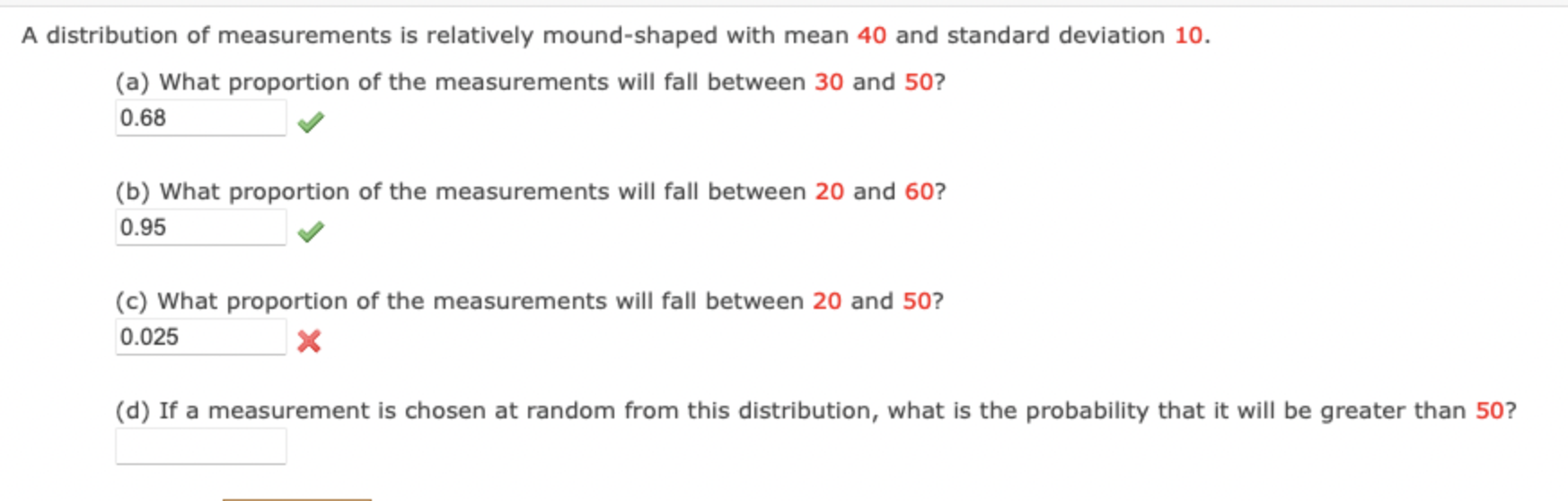# Question Solved1 AnswerA distribution of measurements is relatively mound-shaped with mean 40 and standard deviation 10 . (a) What proportion of the measurements will fall between 30 and 50 ? (b) What proportion of the measurements will fall between 20 and 60 ? (c) What proportion of the measurements will fall between 20 and 50 ? \( x \) (d) If a measurement is chosen at random A distribution of measurements is relatively mound-shaped with mean 40 and standard deviation 10 . (a) What proportion of the measurements will fall between 30 and 50 ? (b) What proportion of the measurements will fall between 20 and 60 ? (c) What proportion of the measurements will fall between 20 and 50 ? \( x \) (d) If a measurement is chosen at random from this distribution, what is the probability that it will be greater than 50 ?J0PVUW The Asker · Probability and Statistics

Hello can u just do question D pleaseTranscribed Image Text: A distribution of measurements is relatively mound-shaped with mean 40 and standard deviation 10 . (a) What proportion of the measurements will fall between 30 and 50 ? (b) What proportion of the measurements will fall between 20 and 60 ? (c) What proportion of the measurements will fall between 20 and 50 ? \( x \) (d) If a measurement is chosen at random from this distribution, what is the probability that it will be greater than 50 ?
More
Transcribed Image Text: A distribution of measurements is relatively mound-shaped with mean 40 and standard deviation 10 . (a) What proportion of the measurements will fall between 30 and 50 ? (b) What proportion of the measurements will fall between 20 and 60 ? (c) What proportion of the measurements will fall between 20 and 50 ? \( x \) (d) If a measurement is chosen at random from this distribution, what is the probability that it will be greater than 50 ?